GeeksforGeeks App
Open AppBrowser
Continue

# Logical Gates using TensorFlow – OR, AND, NOR, XOR, XNOR

TensorFlow provides mathematical operations and logical gates with tensorflow.math module. As input, it requires a tensor. For boolean operations, we can use boolean values True, and False in Tensor.

```Parameters:
A: A Tensor of boolean value.
B: A Tensor of  boolean value.
name (optional): The name for the operation.

For OR GATE
Syntax: tensorflow.logical_or(A, B, name=None) or tensorflow.math.logical_or(A, B, name=None)

For AND GATE
Syntax: tensorflow.logical_and(A, B, name=None) or tensorflow.math.logical_and(A, B, name=None)

For NOT GATE
Syntax: tensorflow.logical_not(A, name=None) or tensorflow.math.logical_not(A, name=None)

For XOR GATE
Syntax: tensorflow.math.logical_xor(A, B, name=None)```

### OR Gate using TensorFlow:

In OR Gate, If one or both the inputs are High(True) then the output will be high(True) else low(False).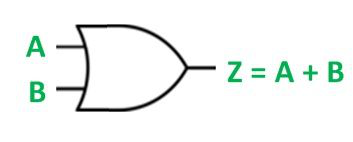OR Gate

## Python3

 `import` `tensorflow as tf`` ` `# Define the input tensors for the OR gate``A ``=` `tf.constant([``False``, ``False``, ``True``, ``True``], dtype``=``tf.``bool``)``B ``=` `tf.constant([``False``, ``True``, ``False``, ``True``], dtype``=``tf.``bool``)`` ` `# Implement the OR gate using the TensorFlow logical_or function``Z ``=` `tf.math.logical_or(A, B)`` ` `# Print the output tensor``print``(Z)`

Output:

`tf.Tensor([False  True  True  True], shape=(4,), dtype=bool)`

Here, we have used tf.math.logical_or() function to implement the logical OR gate. It takes A and B tensors as input and produces output tensor Z.

### AND Gate using TensorFlow:

In AND Gate, If both the inputs are High(True) then the output will be high(True) else low(False).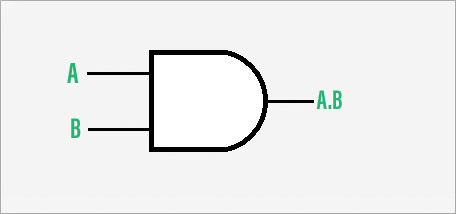AND Gate

## Python3

 `import` `tensorflow as tf`` ` `# Define the input tensors for the AND gate``A ``=` `tf.constant([``False``, ``False``, ``True``, ``True``], dtype``=``tf.``bool``)``B ``=` `tf.constant([``False``, ``True``, ``False``, ``True``], dtype``=``tf.``bool``)`` ` `# Implement the AND gate using the TensorFlow logical_and function``Z ``=` `tf.math.logical_and(A, B)`` ` `# Print the output tensor``print``(Z)`

Output:

`tf.Tensor([False False False  True], shape=(4,), dtype=bool)`

Here, we have used tf.math.logical_and() function to implement the logical AND gate. It takes A and B tensors as input and produces output tensor Z.

### NOT Gate using TensorFlow:

In NOT Gate, The output will be opposite to the input value. i.e If the input is High then the output will be Low and vice versa.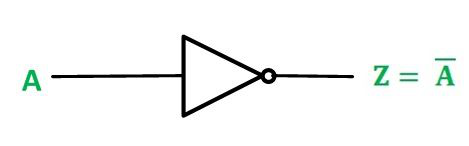NOT Gate

## Python3

 `import` `tensorflow as tf`` ` `# Define the input tensors for the NOT gate``A ``=` `tf.constant([``False``, ``True``], dtype``=``tf.``bool``)`` ` `# Implement the NOT gate using the TensorFlow logical_not function``Z ``=` `tf.math.logical_not(A)`` ` `# Print the output tensor``print``(Z)`

Output:

`tf.Tensor([ True False], shape=(2,), dtype=bool)`

Here, we have used tf.math.logical_not() function to implement the logical NOT gate. It takes A  tensor as input and produces output tensor Z.

### XOR Gate using TensorFlow:

In XOR Gate, If any one of the inputs is high then the output will be high and if both the inputs are high then the output will be low.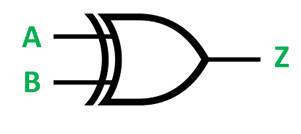XOR Gate

## Python3

 `import` `tensorflow as tf`` ` `# Define the input tensors for the XOR gate``A ``=` `tf.constant([``False``, ``False``, ``True``, ``True``], dtype``=``tf.``bool``)``B ``=` `tf.constant([``False``, ``True``, ``False``, ``True``], dtype``=``tf.``bool``)`` ` `# Implement the XOR gate using the TensorFlow logical_xor function``Z ``=` `tf.math.logical_xor(A, B)`` ` `# Print the output tensor``print``(Z)`

Output:

`tf.Tensor([False  True  True False], shape=(4,), dtype=bool)`

Here, we have used tf.math.logical_xor() function to implement the logical XOR gate. It takes A and B tensors as input and produces output tensor Z.

### NOR Gate using TensorFlow:

In NOR Gate, It provides high output only if all the inputs are low otherwise low output.

Tensorflow does not have a pre-defined logical function for the NOR gate. Therefore, we will be using the logical OR function and logical NOT function in combination to construct a NOR gate.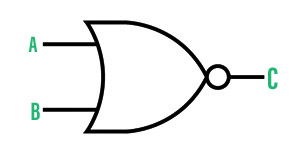NOR Gate

## Python3

 `import` `tensorflow as tf`` ` `# Define the input tensors for the OR gate``A ``=` `tf.constant([``False``, ``False``, ``True``, ``True``], dtype``=``tf.``bool``)``B ``=` `tf.constant([``False``, ``True``, ``False``, ``True``], dtype``=``tf.``bool``)``   ` `# Implement the NOR gate using the``# TensorFlow logical_or function and logical_not``# First implement OR Gate between A and B``Z ``=` `tf.math.logical_or(A, B)`` ` `# Now implement NOT Gate to output of OR GATE i.e 'Z'``C ``=` `tf.math.logical_not(Z)`` ` `# Print the output tensor``print``(C)`

Output:

`tf.Tensor([ True False False False], shape=(4,), dtype=bool)`

Here, we have used tf.math.logical_or() function to implement the logical OR gate. It takes A and B tensors as input and produces output tensor Z. and the we have used tf.math.logical_not() function to implement the logical NOT gate. It takes output tensor of OR gate as input and produces output tensor C. This combined result is output of NOR Gate

### XNOR Gate using TensorFlow:

In XNOR Gate, The output will be high only if both the inputs will be the same i.e either High or Low.

Similar to the NOR gate, Tensorflow does not have a function for the XNOR gate. Thus, we will combine logical XOR and logical NOT functions to construct the XNOR gate.XNOR Gate

## Python3

 `import` `tensorflow as tf`` ` `# Define the input tensors for the XOR gate``A ``=` `tf.constant([``False``, ``False``, ``True``, ``True``], dtype``=``tf.``bool``)``B ``=` `tf.constant([``False``, ``True``, ``False``, ``True``], dtype``=``tf.``bool``)`` ` `# Implement the XNOR gate using the``# TensorFlow logical_xor function and logical_not``# First implement OR Gate between A and B``Z ``=` `tf.math.logical_xor(A, B)``# Now implement NOT Gate to output of XOR GATE i.e 'Z'`` ` `Y ``=` `tf.math.logical_not(Z)``# Print the output tensor``print``(Y)`

Output:

`tf.Tensor([ True False False  True], shape=(4,), dtype=bool)`

Here, we have used tf.math.logical_xor() function to implement the logical OR gate. It takes A and B tensors as input and produces output tensor Z. and we have used tf.math.logical_not() function to implement the logical NOT gate. It takes the output tensor of XOR gate as input and produces the output tensor Y. This combined result is the output of XNOR Gate.